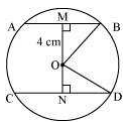Guru

# The lengths of two parallel chords of a circle are 6 cm and 8 cm. If the smaller chord is at a distance 4 cm from the centre, what is the distance of the other chord from the centre? Q.3

• 0

How i find the best tricky way for solving the question of class 9th of Circles chapter of class 9th give me the best and easy method for this question no.3 of exercise 10.6 The lengths of two parallel chords of a circle are 6 cm and 8 cm. If the smaller chord is at a distance 4 cm from the centre, what is the distance of the other chord from the centre?

Share

1. Consider the following diagramHere AB and CD are 2 parallel chords. Now, join OB and OD.

Distance of smaller chord AB from the centre of the circle = 4 cm

So, OM = 4 cm

MB = AB/2 = 3 cm

Consider ΔOMB

OB2 = OM2+MB2

Or, OB = 5cm

Now, consider ΔOND,

OB = OD = 5 (since they are the radii)

ND = CD/2 = 4 cm

Now, OD2= ON2+ND2

Or, ON = 3 cm.

• 0